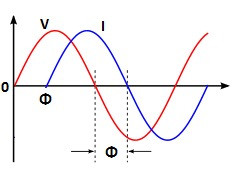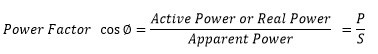Search
• Raj

# Displacement Power Factor, Distortion Power Factor & True Power Factor

Updated: Mar 2

## What is Displacement Power Factor?

There are three power factor terms widely used for electrical network. The terms are rather confusing and its clear understanding is must for an electrical engineer. We shall discuss in detail the three terms of power factor in this post.

1. Displacement Power Factor

2. Distortion power factor

3. True Power Factor

### Displacement Power Factor

When linear loads are connected to sinusoidal voltage source, the current drawn by the load is also sinusoidal. The current may be in phase with the applied voltage or in out of phase with the applied voltage. The phase displacement depends on nature of load. The linear devices are the devices which follow Ohm's law. Inductor, capacitor and resistance are the linear devices and the current drawn by these devises is in linear relationship with the applied voltage.The cosine of the phase angle difference ( CosΦ) between voltage and current is called the displacement power factor(DPF) or fundamental power factor.

When AC voltage is fed to resistive,inductive and capacitive load, the current flowing through these components has different phase angle shift with respect to the applied voltage. Current and voltage are in phase for resistive load. The resistive load draws only active power. It does not draw reactive power. The reactive power is wattless current. In the case of pure inductive and capacitive load the current is reactive in nature. Thus in AC circuit, three power terms are used.

1. Active or real power

2. Reactive Power

3. Apparent Power

The load triangle of three power is as given below.The ratio of real or active power to apparent power is known as power factor.The power factor of the resistive load is unity because reactive power drawn by resistive load is zero. The apparent power is vector sum of the real power and reactive power.The power factor of the system depends on the inductive load. The more inductive load draws large reactive power and thus the total apparent power increase and cause low power factor.

## What is Distortion Power Factor?

In older days, most of the loads were linear loads. However, after advanced development in power semiconductor devices, every industry now using the variable frequency drives,DC drives, inverters,converters and many electronics equipment for ease of operation and power saving.

The semiconductor devices like power diodes, MOSFET,IGBTs are non linear types of load and the current drawn by these devices does not vary according to voltage. These devices does not follow the Ohm's law and called non ohmic or non linear devices.

The semiconductor devices draw reactive power from the source on account of harmonics produced by devices. The current drawn by semiconductor device has harmonics. The integral multiples frequency of the fundamental frequency is called harmonics. Thus, these devices pollutes the electrical power network. The harmonics is type of distortion which does not do any real work and cause drawing of watt-less current from the supply source.

The harmonics can be measured with the help of harmonics analyzer. The total harmonic distortion in voltage and current can be measured with the harmonic analyzer. The total harmonic distortion in voltage can be expressed by following mathematical expression.Similarly, total harmonic distortion in current can be expressed by following mathematical expression.V1 and I1 is the fundamental voltage and current.

The load which has more total harmonic distortion draws more reactive power and distortion power factor is low. The distortion power factor depends on the total harmonic distortion produced by the load.

The distortion power factor can be expressed by following mathematical expression.From above formula, it is clear that higher the THD, lower will be the distortion factor. The power factor deteriorates with increased total harmonic distortion.## What is True Power Factor?

From above discussion, it is clear that overall power factor of the electrical network depends on;

1. Displacement power Factor

2. Distortion power Factor

The true power factor is equal to the product of displacement power factor and distortion power factor.

True Power Factor = Displacement power factor X Distortion power factor

The true power factor of an electrical network can be expressed by following mathematical expression.